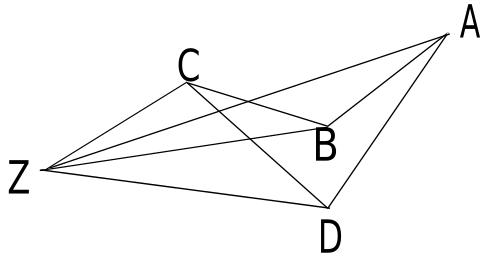### Stable Spinning Incompressible Viscous Fluid

I want to find the ultimate shape of a blob of spinning matter after it has dissipated all of its free internal energy and all the energy available due to internal shear forces, but without losing any of its angular momentum.

Gravity attracts. There are no shear forces, only pure isotropic pressure (which varies from point to point but not from direction to direction). The pressure is zero on the surface. I conjecture that in some reference frame revolving at a uniform angular velocity ω all matter is at rest in the frame. If ω=0 then we have a sphere. For small positive ω we have an oblate spheroid, at least approximately. For some larger ω’s we have two blobs in circular orbits about each other. There may be ω’s for which both configurations are possible. I want to learn the shape of the blobs considering tidal forces. I further conjecture that the shape is unique for given angular momentum L except for the distribution of the two masses in the large ω case.

The plan is to represent that proportion of space occupied by fluid as the union of tetrahedra. “Sum of tetrahedra” would be more accurate in the sense that some tetrahedra make a negative contribution. I illustrate here in 2D.Observe the quadrilateral ABCD which we wish to describe with triangles. You might think of point Z as the origin; it doesn’t matter. There are four triangles, all thru Z, one for each sides of the quadrilateral. Each triangle shares one side with the quadrilateral and has two other sides going to point Z. The triangles overlap. Triangles ABZ and CDZ are both negative while BCZ and DAZ are positive. Outside the quadrilateral the triangles cancel and within they add up exactly once. It is good old fashioned planimeter logic. The same works in 3D.

The plan is to triangulate the one or two surfaces of the blob. For each triangle record the tetrahedron (sliver) formed of that triangle and the origin which is the center of gravity of the blob. Every sliver will have a mass which is its volume; some of which will be negative. We are done when for each component the vertices of the surface of that component are at the same potential. In addition to the gravitational potential is the centrifugal potential which is merely −ω2r2. To progress move a bit of mass from one vertex to another of the same component. This is done by nudging a vertex and adjusting the neighboring slivers. We might include logic to keep the areas of the surface triangles nearly equal. We must keep the blob spinning about its center of mass and with the principle eigenvector of its inertia tensor as its axis. We must also preserve the angular momentum.

It is complex to detect intersecting blobs or even a blob that intersects itself. I hope that my program does not discover that energy can be reduced by self intersecting configurations. If we spin-down a pair of orbiting blobs they will meet at some point and coalesce unless we detect and proscribe that. One could view this as the program exploiting a topological trick to achieve density 2, or even more. I think that every solution with two connected components is separated by some plane.

There is perhaps a worse topological pitfall. Imagine a very oblate blob. Just pushing the surface in for those urface parts with low potential may well lead to the upper and lower surfaces passing each other to form a negative volume region. I imagine adding some surface tension but decreasing it to 0 as we proceed toward a solution.

Here is a high level plan for a computation.